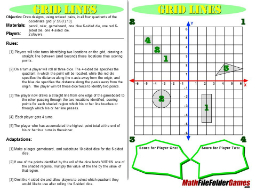# Grid Lines – Coordinate Grid GameDownload this Game FREE and File it Away for Later! (NO EMAIL Opt-in Required)

Grid Lines is a game of chance that depends on the roll of three dice. The red die tells the player how far from the origin his or her point is along the x-axis. The blue die tells the player how far from the origin his or her point is along the y-axis. The third die tells in which quadrant the player’s point will be placed. In a sense, the player is learning the idea of absolute value here. He or she won’t roll a negative number on a die, but the position will be positive or negative from the zero point depending on the quadrant in which the final point will rest.From an educational standpoint, this game is very strong. In a classroom environment, students will have a much more thorough understanding of the coordinate system after experimenting with this game than they ever would just from plotting points that are listed on a text page. You can tie this game to some of the Euclidean Geometry postulates, such as the fact that any two points determine a line. A great introduction to this game would be some basic discussion about absolute value. The fact that a distance is not a negative measure but that the negative is really designating a direction just like north, south, east, west is designated on a map.

Another way to play this game is to create a story. The square that’s labelled “4” with grey tint could designate a buried treasure. If a player rolls the correct die combination to cross into that square, he or she gets the treasure. You’ll have to figure out something clever you can give as a prize!

After several games are played, it would be interesting to compare them to see how often the lines cross over the grey tinted areas. This could lead to a discussion regarding some elementary probability concepts. How frequently would they expect to see a line cross over one of the grey tinted areas?

It might also be interesting to let students use different colored pencils for each round of this game so they can keep track of the moves they made first, second, third. It would give the finished games a colorful, artistic feel. Lots of exploration questions could be posed such as: How often could you expect to see a line that is parallel to the given axes? Can you figure out where the two lines that players played in the same round intersect each other? Another companion idea would be to have students record their die combinations on an x-y table. From this beginning you can start discussing standard notation for coordinate points and lines in the plane. You can also begin an elementary discussion of absolute value.

This game is also available as a iOS APP, read more about the app hereDownload this Game FREE and File it Away for Later! (NO EMAIL Opt-in Required)

#### Common Core Mathematical Standards

5.G.1 Use a pair of perpendicular number lines, called axes, to define a coordinate system, with the intersection of the lines (the origin) arranged to coincide with the 0 on each line and a given point in the plane located by using an ordered pair of numbers, called its coordinates. Understand that the first number indicates how far to travel in the direction of the second axis, with the convention that the names of the two axes and the coordinates correspond (e.g., x-axis and x-coordinate, y-axis and y-coordinate).
5.G.2 Represent real world and mathematical problems by graphing points in the first quadrant of the coordinate plane, and interpret coordinate values of points in the context of the situation.
6.G.3 Draw polygons in the coordinate plane given coordinates for the vertices; use coordinates to find the length of a side joining points with the same first coordinate or the same second coordinate. Apply these techniques in the context of solving real-world and mathematical problems.
6.NS.6 Understand a rational number as a point on the number line. Extend number line diagrams and coordinate axes familiar from previous grades to represent points on the line and in the plane with negative number coordinates.
6.NS.6.b Understand signs of numbers in ordered pairs as indicating locations in quadrants of the coordinate plane; recognize that when two ordered pairs differ only by signs, the locations of the points are related by reflections across one or both axes.
6.NS.6.c Find and position integers and other rational numbers on a horizontal or vertical number line diagram; find and position pairs of integers and other rational numbers on a coordinate plane.
6.NS.8 Solve real-world and mathematical problems by graphing points in all four quadrants of the coordinate plane. Include use of coordinates and absolute value to find distances between points with the same first coordinate or the same second coordinate.## BEST SELLING MIDDLE SCHOOL MATH GAMESGet the above math game PLUS 41 more fun middle school (& upper elementary) math games to use with your students.